# GMAT Math : Calculating the surface area of a cube

## Example Questions

2 Next →

### Example Question #11 : Calculating The Surface Area Of A Cube

Aperture labs makes a variety of cubes. If each cube has a volume of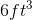, what is the surface area of the cube?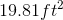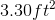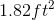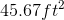Explanation:

Aperture labs makes a variety of cubes. If each cube has a volume of, what is the surface area of the cube?

Let's work backwards from our goal in this question.

We know that we need to find surface area. To find surface area of a cube, we can use the following equation: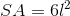Where l is the length of one side.

Next, let's look at the volume formula: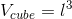So, we can find our length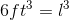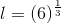Let's leave l like that for the moment, and use it to find our surface area.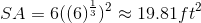2 Next →

Tired of practice problems?

Try live online GMAT prep today.